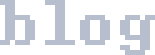skip nav

### A Variadic println() for Arduino

The Arduino ecosystem provides `Serial.print(x);` and `Serial.println(x);`, the latter adding a newline after the value. I almost universally want to print out out a tag so I know what's what though, so something like `Serial.printlnMulti(x, y, z, ...)` would be convenient. (`x`, `y`, and `z` and so on can be any type here.)

And indeed, we can make it so.

debug.hpp: ``` #pragma once #include <Arduino.h> template <class ...Types> void debug(Types&& ...inputs) { (Serial.print(inputs), ...); Serial.println(); } ```

Usage like `debug("analog value: ", analogRead(35));`. You can have any number of args to the function, of course.

What are the size implications of this, however? C++ is big on zero-cost abstractions, so ideally the above should copile the same as:

``` Serial.print("analog value: "); Serial.println(analogRead(35)); ```

Testing this in my non-trivial project, substituting one debug stanza of many, we get a total size for the fancy C++ 17 version, 279957 bytes. This compares favourably to the baseline of 279973 bytes, as we have paid a total of -20 bytes for our indescretions. A win for expressing what you want over expresning how to do it, I guess. ¯\_(ツ)_/¯

tags: arduino, embedded, code